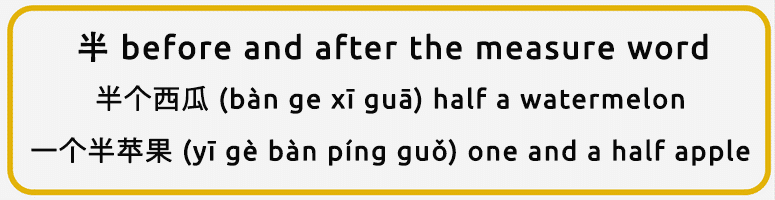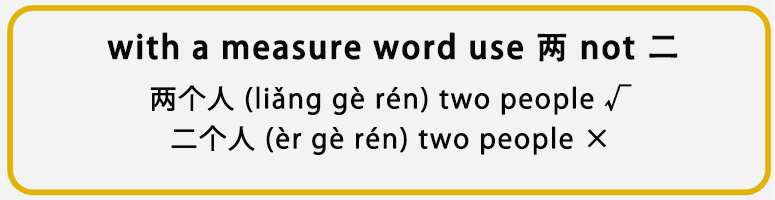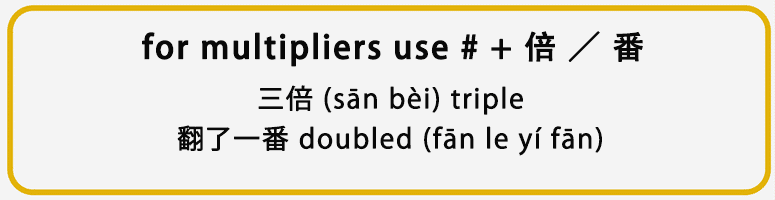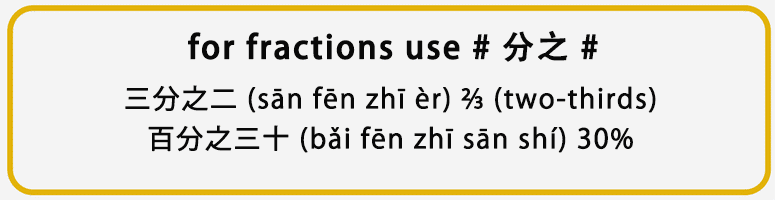﻿ The Practical Uses of Chinese Numerals The Practical Uses of Chinese Numerals

Use this tool to add tone marks to pinyin or to convert tone number (e.g. hao3) to tone marks.

Although you can use the red buttons to add tone marks, we highly recommend you use the number method (e.g. hao3) for speed and placement of the accent above the correct vowel. [Hint: Type "v" for "ü"]
Note: You do not need to use this tool to enter pinyin in this dictionary.

## The Practical Uses of Chinese NumeralsIt will only take 7 minutes to read this post!

You’ve probably already learned the basic numbers in Chinese, but there are ways in which Chinese numerals are used in specific ways that are different to in English. You’ll learn some of these differences, as well as some practical ways to use Chinese numerals for discounts, fractions and to make approximations.

## 1. 基数词 (jī shù cí) Cardinal numerals and 序数词 (xù shù cí) Ordinal numbers

### a. 基数词 Cardinal numbers include the following:

• 系数词 (xì shù cí) constant numbers: 零 (líng), 半 (bàn), 一 (yī), 二 (èr) 两 (liǎng, 三 (sān), 四 (sì), 五 (wǔ) 六 (liù), 七 (qī), 八 (bā), 九 (jiǔ), 十 (shí)。
• 位数词 (wèi shù cí) digit numbers: 十 (shí), 百 (bǎi) 千 (qiān), 万 (wàn), 亿 (yì)。
• 复合数词 (fù hé shù cí) compound numerals: 十五 (shí wǔ), 八十 (bā shí), 九千 (jiǔ qiān), 十亿 (shí yì)。

When ‘zero’ 零 (líng) is used between constant or digital numbers it is only added once, for example:

30050 = 三万零五十

50006 = 五万零六

(bàn) can be put both before and after the measure word.For example:

There is one exception when 半 (bàn) is found next to a numeral, in the chengyu 年过半百 (nián guò bàn bǎi. In this situation, 半百 (bàn bǎi) means ‘50 years old’.

In Chinese, numbers can be divided into “个 (gè), 十 (shí), 百 (bǎi), 千 (qiān) and 万 (wàn)” (a/an, 10, 100, 1000, 10000). Digits are grouped into 4’s and all numbers over “万” 10000, use 万 as the unit; numbers over 亿 1000000000, use 亿 as a unit.

For example:

5726，4832，9803 = 五千七百二十六亿，四千八百三十二，九千八百零三

1. ### b. 序数词 (xù shù cí) Ordinal numbersFor example:

(èr) and (liǎng) both mean number ‘2’, but the usages are different:

• 二 (èr) can be used as “the second” without 第 (dì), whereas 两 (liǎng) can not be used as an ordinal number.

For example:

One exception is that 两 (liǎng) used to say 2 o’clock: 两点钟 (liǎng diǎn zhōng).

Generally, when used before measure words, we will use 两 (liǎng), not 二 (èr).For example:

An exception is before Chinese traditional meteorological measure words, when both 二 (èr) and 两 (liǎng) can be used.

For example:

• 两 (liǎng) can be used as an ‘approximate’ number, which is similar to (jǐ).

For example:

## 2. 倍数 (bèi shù) Multipliers, 分数 (fēn shù) Fractions and 概数 (gài shù) Approximate numbers

### a. 倍数 (bèi shù) Multipliers

Common use: 倍数 (bèi shù) = # + (bèi) / (fān).For example:

For example, you can say 增加了三倍 (zēng jiā le sān bèi) ‘to increase 3 times’, but you can not say 减少了三倍 (jiǎn shǎo le sān bèi) ‘to reduce 3 times’ ×.

1. ### b. 分数 (fēn shù) Fractions

Common use: 分数 (fēn shù)= # 分之 #In spoken Chinese, (chéng) or (fēn) can be used as #/10, for example:

In addition, when talking about discounts, we will use # + (zhé), for example:1. ### c. 概数 (gài shù) Approximate numbers

Common use: 概数 (gài shù) = # + 多 (duō) / 来 (lái) / 左右 (zuǒ yòu) / 上下 (shàng xià)We can also use two consecutive numbers as 概数 (gài shù) an approximate number.

For example:

Generally, the smaller number should be put before the bigger. However, there is an exception with 三两 (个) (sān liǎng (gè)) , which emphasises the number is “few”.

There are two exceptions that don’t use consecutive numbers to indicate an approximation:

In addition, some commonly used words can be used as 概数 (gài shù) approximate numbers. For example, 若干 (ruò gān) ‘how many’ and 好些 (hǎo xiē) ‘quite a lot’.

1. ### 3. Some Further examples

Numerals are always used with measure words with a noun, or to emphasise a specific number.

For example:

Generally, in spoken Chinese, numerals must be used with a measure word before a noun. But in written Chinese, you can use a numeral with a noun.

For example:

Examples here are not meant to be an exact number, but are abstract to mean “many” or “few”.•Anup Pokhrel says: